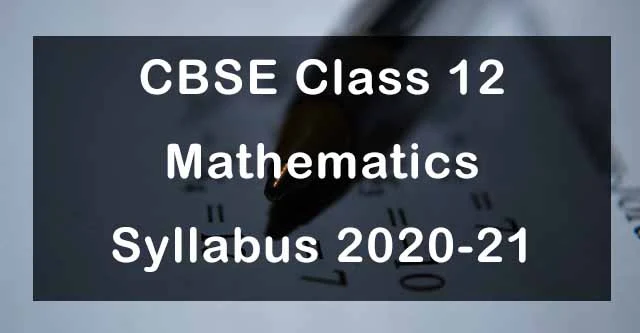## CBSE Class 12 Maths Syllabus 2020-21| Check Latest Exam Pattern

CBSE has released Class 12 Maths Syllabus 2020-21 that are very useful in understanding the exam pattern and important topics. Due to ongoing health crisis, the board has also decided to remove some topics to ease the burden. The question paper will be of 80 marks while internal assessment will be given 20 marks.S. No. Units Marks I Relations and Functions 08 II Algebra 10 III Calculus 35 IV Vectors and Three - Dimensional Geometry 14 V Linear Programming 05 VI Probability 08 - Internal Assessment 20 Total 100

Unit-I: Relations and Functions

1. Relations and Functions

Types of relations: reflexive, symmetric, transitive and equivalence relations. One to one and onto
functions.

2. Inverse Trigonometric Functions

Definition, range, domain, principal value branch.

Unit-II: Algebra

1. Matrices

Concept, notation, order, equality, types of matrices, zero and identity matrix, transpose of a matrix, symmetric and skew symmetric matrices. Operation on matrices: Addition and multiplication and multiplication with a scalar. Simple properties of addition, multiplication and scalar multiplication. Noncommutativity of multiplication of matrices, Invertible matrices; (Here all matrices will have real entries).

2. Determinants

Determinant of a square matrix (up to 3 x 3 matrices), minors, co-factors and applications of determinants in finding the area of a triangle. Adjoint and inverse of a square matrix. solving system of linear equations in two or three variables (having unique solution) using inverse of a matrix.

Unit-III: Calculus

1. Continuity and Differentiability

Continuity and differentiability, derivative of composite functions, chain rule, derivative of inverse trigonometric functions, derivative of implicit functions. Concept of exponential and logarithmic functions.

Derivatives of logarithmic and exponential functions. Logarithmic differentiation, derivative of functions expressed in parametric forms. Second order derivatives.

2. Applications of Derivatives

Applications of derivatives: increasing/decreasing functions, tangents and normals, maxima and minima (first derivative test motivated geometrically and second derivative test given as a provable tool). Simple problems (that illustrate basic principles and understanding of the subject as well as reallife situations).

3. Integrals

Integration as inverse process of differentiation. Integration of a variety of functions by substitution, by partial fractions and by parts, Evaluation of simple integrals of the following types and problems based on them.Fundamental Theorem of Calculus (without proof).Basic properties of definite integrals and evaluation of definite integrals.

4. Applications of the Integrals

Applications in finding the area under simple curves, especially lines, parabolas; area of circles /ellipses (in standard form only) (the region should be clearly identifiable).

5. Differential EquationsUnit-IV: Vectors and Three-Dimensional Geometry

1. Vectors

Vectors and scalars, magnitude and direction of a vector. Direction cosines and direction ratios of a vector. Types of vectors (equal, unit, zero, parallel and collinear vectors), position vector of a point, negative of a vector, components of a vector, addition of vectors, multiplication of a vector by a scalar, position vector of a point dividing a line segment in a given ratio. Definition, Geometrical Interpretation, properties and application of scalar (dot) product of vectors, vector (cross) product of vectors.

2. Three - dimensional Geometry

Direction cosines and direction ratios of a line joining two points. Cartesian equation and vector equation of a line, coplanar and skew lines, shortest distance between two lines. Cartesian and vector equation of a plane. Distance of a point from a plane.

Unit-V: Linear Programming

1. Linear Programming

Introduction, related terminology such as constraints, objective function, optimization, different types of linear programming (L.P.) problems. graphical method of solution for problems in two variables, feasible and infeasible regions (bounded), feasible and infeasible solutions, optimal feasible solutions (up to three non-trivial constraints).

Unit-VI: Probability

1. Probability

Conditional probability, multiplication theorem on probability, independent events, total probability, Bayes’ theorem, Random variable and its probability distribution.

Prescribed Books:

• Mathematics Part I - Textbook for Class XII, NCERT Publication
• Mathematics Part II - Textbook for Class XII, NCERT Publication